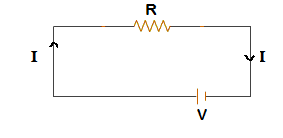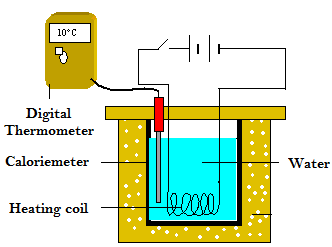# Derivation of Joules’s Law of Heating

When current is passed through a resistance some work is done.In a passive resistance the work done is equal to the amount of heat evolved in a resistance.Consider a battery having terminal p.d ‘V’ is connected with a resistance as shown in figure. Let ‘I’ be the amount of current in the circuit. Let ‘q’ be the charge passed through the resistance in time‘t’ second.

We  know that,

$\text{Current (I)} = \frac{\text{Charge (q)}}{\text{Time (t)}}$

$\text{q = It}……(i)$

If the potential difference between A and B is V. Then,

$\text{V = IR}……(ii)$

Let ‘W’ be the amount of work done to move ‘q’ amount of charge through the resistance.

$\text{ So, Electric potential(V)} = \frac{\text{Workdone (W)}}{\text{q }}$

$\text{ or, W = qV}$

$\text{ W=VIt}……(ii)$

Now, from equation (i) and (ii)  and (iii),

$\text{W = It.IR}$

$\text{or, W = I}^2 \text{ RT}$

Heat produced, H= RT  joule

In CGS system,

$\text{H} = \frac{\text{I}^2\text{RT}}{\text{J}}\text{Cal}$$Verification of Joule’s law:Apparatus setup for the verification of Joule’s Law of heating Take a glass container containing some amount of water (about 1/3rd). Take a coil, dip it into the water and connect the coil in a circuit containing a cell, ammeter, rheostat and switch as shown in figure. Thermometer is dipped into the water to measure the temperature of water. As we pass current, the coil will be heated and water absorb that amount of heat. ∴ The amount of heat produced by the coil, H = heat absorbed by the water. or, H=ms(θ2 – θ1) Here, θ1 and θ2 are initial and final temperature of water. ‘m’ & ‘s’ are the mass and specific heat capacity of water respectively. 1. Verification of H∝I2 Dip a coil into the water and pass certain amount of current for certain time(say 5 minute).Then calculate the amount of heat produced by the coil by using H=ms(θ2 – θ1) By the help of the rheostat, pass different amount of current in the coil for the same time (say 5 minute) and calculate the heat produced. Let H1, H2 and H3 be the amount of heat produced for current I1, I2 and I3 respectively. Here, we find,$\frac{H_1}{I_1^2} =  \frac{H_2}{I_2^2} = \frac{H_3}{I_3^2}\therefore   \frac{H}{I^2} = \text{Constant}$On plotting the graph between H and I2 we get a straight line passing through origin.$\text{So, we can conclude that, }H  \propto I^2$2. Verification of H∝R Pass certain amount of current for certain time (say 5 minute) through different coil and calculate the different amount of heat produced by them as before. Let be the amount of heat produced by the resistance respectively. Here, we find,$\frac{H_1}{R_1} =  \frac{H_2}{R_2} = \frac{H_3}{R_3}\therefore   \frac{H}{R} = \text{Constant}$On plotting graph between H and R,we get a straight line passing through origin.$\text{So, we can conclude that, }H  \propto R$3. Verification of H∝T Pass certain amount of current through a coil for different time and find the amount of heat produced by the coil as before. Let H1, H2 and H3 be the amount of heat produced by the coil in time T1, T2 and T3 respectively. Here, we find:$\frac{H_1}{T_1} =  \frac{H_2}{T_2} = \frac{H_3}{R_3}\therefore   \frac{H}{T} = \text{Constant}$On plotting a graph between H and T, we get straight line passing through origin.$\text{So, we can conclude that, }H  \propto T\$

Hence, Joule’s law of heating is verified.

Do you like this article ? If yes then like otherwise dislike : 19

#### One Response to “Derivation of Joules’s Law of Heating”

1.Manisha

Derivation of joules law